# Numerical Study of Thermal Convection in a Porous Medium

Numerical Study of Thermal Convection in a Porous Medium
Laboratory of Applied Energy Physics, Department of Physics, Faculty of Sciences of Matter, Batna 1University, Batna Algeria

Corresponding Author Email:
azeddine.soudani@univ-batna.dz
Page:
69-74
|
DOI:
https://doi.org/10.18280/i2m.180111
18 December 2018
|
Accepted:
5 February 2019
|
Published:
20 April 2019
| Citation

OPEN ACCESS

Abstract:

This study is dealing with the numerical simulation of natural convection, in a cubic shaped cavity. The surrounding walls are partially porous, homogeneous, and isotropic. The horizontal walls are adiabatic. The vertical walls of the cavity are maintained at constant temperatures (Dirichlet's boundary conditions), and the fluid is the air. The flow in the porous environment is modeled by Darcy-Brinkman-forchheimer model. The finite volumes are used for the resolution of the equation of continuity, momentum and energy. The obtained results were reported to the following: Rayleigh number, Darcy number, and dimensionless thickness of the porous layer and the Nusselt number.

The obtained results show that the increase is proportional to the increase of Rayleigh and Darcy numbers and proportionally inverted with dimensional thickness of the porous layer.

Keywords:

Porous media, natural convection, cubic cavity, finite volume method

1. Introduction

Fluid flows through a porous medium are frequently encountered in both nature and industry. The fields of application are many and varied, we can mention the problems of purification of water, soil remediation, oil and gas extraction, etc. For this, the transfers in saturated porous media have been the subject of numerous theoretical and experimental work. Because of their importance in many areas.

Natural convection in porous media has been a topic of research for many authors, such as:

- In 2005, Sami Ben Amara did a work on natural convection flows and heat transfer in macro-porous food environments: application to refrigerators. The approach is both experimental and numerical .

- Yacine Ould-Amer et al. are interested in natural convection in a porous multilayer square cavity. Each layer of porous medium is considered homogeneous, isotropic and saturated by a single fluid .

- For a numerical and analytical study of the natural convection heat transfer in porous layers to optimize the effect of the Darcy drag, R. Rebhi et al. proposed the following model: a horizontal rectangular cavity subjected to a Vertical thermal gradient with transition flow governing a single-cell or multi-cell convective flux .

- F. Habbachi et al. are interested in the three-dimensional simulation of natural convection in a cubic cavity partially filled with porous media .

In this study, we are interested in the heat and mass transfer induced by natural convection in a partially porous cavity. It is a question of studying the influence of the control parameters on the flow and the mechanisms of heat transfer. The vertical walls of the entire domain are subjected to constant temperatures. The Darcy-Brinkman-Forcheimer formulation in anisotropic saturated permeable porous medium has been retained in the present work.

2. Mathematical Formulation

The physical model studied is shown in Figure 1. It is a cubic cavity of geometrical parameter (H), partially filled with a porous layer of thickness e, and saturated by a single fluid. We call η=e / H the dimensionless thickness of the porous layer.

Vertical surfaces are subjected to Dirichlet temperature conditions, while horizontal surfaces are adiabatic (Neumann conditions).

## 1.pngFigure 1. Geometry of the problem

Some approximations have been considered to simplify the formulation of the mathematical model. We limit ourselves to the hypotheses commonly used in natural convection studies which are: The fluid is Newtonian, incompressible and satisfies the Boussinesq hypothesis; the flow of fluid within the cavity is laminar and three-dimensional; it is assumed that the porous matrix is isotropic, homogeneous and in thermodynamic equilibrium with the fluid; the thermo-physical properties of the fluid are constant in the temperature range studied; and we neglect the energy transfer by radiation and by conduction.

In this study, we will therefore adopt the single-domain approach of writing a single equation for the whole domain (Navier Stokes including the term Darcy), and the transition from the porous medium to the fluid medium is done by a variation of permeability ; Navier Stokes equations including the terms Darcy-Brinkman and Forchheimer. Given the assumptions made previously, the classical conservation equations are as follows:

• The continuity equation:

$\frac{∂u}{∂x}+\frac{∂v}{∂y}+\frac{∂w}{∂z}=0$ (1)

• The amount of motion equations is following:

$ρ_f\frac{1}{ε}\frac{∂u}{∂t}+\frac{1}{ε^2}(\overrightarrow{V}.\overrightarrow{∇})u)=-\frac{∂p}{∂y}+μ_{eff} ∇^2 u-\frac{μ}{K}u-\frac{ρ_f}{\sqrt{k}}F|\overrightarrow{V}|u$ (2)

• The amount of motion equations is following:

$ρ_f(\frac{1}{ε}\frac{∂v}{∂t}+\frac{1}{ε^2}(\overrightarrow{V}.\overrightarrow{∇})v)=-\frac{∂p}{∂y}+μ_eff ∇^2 v- \frac{μ}{K} v-\frac{ρ_f}{\sqrt{k}} F|\overrightarrow{V}|v$ (3)

• The amount of movement following equation z:

$ρ_f (\frac{1}{ε} \frac{∂w}{∂t}+\frac{1}{ε^2} (\overrightarrow{V}.\overrightarrow{∇})w)=-\frac{∂p}{∂z}+μ_eff ∇^2 w- \frac{μ}{K} w-\frac{ρ_f}{\sqrt{k}}F|\overrightarrow{V}|w+ρ_f \overrightarrow{g}$ (4)

• Energy equation in porous media:

$(ρc)_m \frac{∂T}{∂t}+{(ρc)}_f (\overrightarrow{V}.\overrightarrow{∇})T=\overrightarrow{∇}.(K_eff .\overrightarrow{∇}T)$ (5)

The equations of the problem are made dimensionless with the following guideline values:

$X=\frac{x}{H}$; $Y=\frac{y}{H}$; $Z=\frac{z}{H}$; $U=\frac{uH}{a}$; $V=\frac{vH}{a}$; $W=\frac{wH}{a}$;

$η=\frac{e}{H}$; $θ=((T-T_0)⁄∆T)$

So the system of equations defining the movement is then written as follows dimensionless:

• The continuity equation:

$\frac{∂U}{∂X}+\frac{∂V}{∂Y}+\frac{∂W}{∂Z}=0$(6)

• The amount of equation of motion according to X:

$(ε\frac{∂U}{∂t}+(\overrightarrow{V}.\overrightarrow{∇})U)=-\frac{∂P}{∂X}+εR_v P_r ∇^2 U- ε^2 (\frac{P_r}{Da}U-\frac{{Fε}^2}{\sqrt{Da}} |\overrightarrow{V}|U$ (7)

• The following movement amount of the equation Y:

$(ε\frac{∂V}{∂t}+(\overrightarrow{V}.\overrightarrow{∇})V)=-\frac{∂P}{∂Y}+εR_v P_r ∇^2 V-ε^2 (\frac{P_r}{Da}V -\frac{{Fε}^2}{\sqrt{Da}}|\overrightarrow{V}|V$ (8)

• The amount of movement in Z equation:

$(ε \frac{∂W}{∂t}+(\overrightarrow{V}.\overrightarrow{∇})W)=-\frac{∂P}{∂Z}+εR_v P_r ∇^2 W-ε^2 (\frac{P_r}{Da})W -\frac{{Fε}^2}{\sqrt{Da}} |\overrightarrow{V|}W$

$+ε^2 R_a.P_r θ$ (9)

• Equation porous medium energy:

$σ \frac{∂θ}{∂t}+((\overrightarrow{V}.\overrightarrow{∇})θ=R_K ∇^2 θ$ (10)

With: RD: mass diffusivity Report; Rk: thermal conductivity ratio;

RV: viscosity of the Report the term Brinkman; β: the coefficient of thermal volumetric expansion of the fluid.

Or:   ρf0 (1-β∆T)

Turning the dimensionless form of the conservation equations shows numbers without the characteristic dimensions of the problem. These parameters are:

 Types Expressions Le nombre de Prandtl $Pr= \frac{ν}{a}$ Le nombre de Grashof $Gr= \frac{gβ∆TL^3}{ν^2}$ Le nombre de Rayleigh $Ra= \frac{gβ∆TL^3}{νa}$= Gr.Pr. Le nombre de Darcy $Da= \frac{K}{e^2}$ l’épaisseur adimensionnelle de la couche poreuse. η=$\frac{e}{H}$

In the end, we express heat transfer on the active surfaces of the dimensionless Nusselt number (Nu) defined by:

$\overrightarrow{Nu}=(h.L)⁄K_f =-(a(K_{eff}-1)+1) \frac{∂T}{∂x}$ (11)

Ḱ= Keff/Kf; Keff=Ks (1-ε) +εKf

The initial and boundary conditions corresponding to this problem are:

Table 2. Terms speed and temperature

## 2.png3. Results and Discussions

In this part, we will present the results obtained by fluent of natural convection in laminar regime in a closed cubic cavity and partially porous. Calculations will allow to show the influence of the number of Rayleigh (Ra) and the permeability (η, Da) on the thermal and dynamic fields.

And for a better accuracy of the results, the choice of an optimal mesh is not random. The independence of the mesh is a simulation test carried out with an increasingly refined mesh until the solution no longer varies with the refinement of the mesh. The choice of geometry is shown in Figure 2.

## 2tu_.png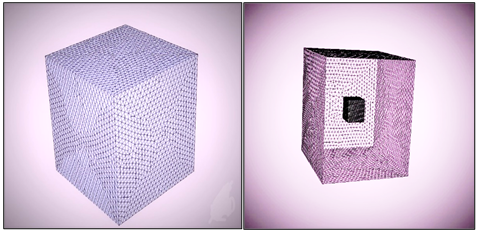Figure 2. Hybrid mesh of tetrahedral form

And Figure 3, shows the temperature and speed profiles as a function of the horizontal position. We can notice that the profiles are identical, so we can choose a mesh that does not take much time to analyze the maximum cases in our study. Our choice was oriented towards the mesh of 15625 nodes.

## 3.png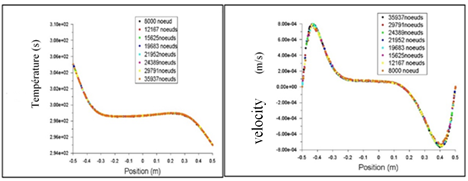Figure 3. Mesh test

The simulation by the software "Fluent" was realized, and the results obtained are compared with the results of F. Habbachi .

- Isotherms, velocity vectors and current lines:

## 4.pngFigure 4. Thermal and dynamic fields for η=20%;

(a) Present study and (b) F. Habbechi 

## 5.png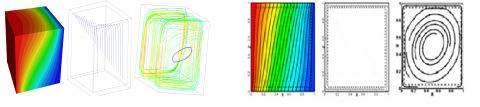Figure 5. Thermal and dynamic fields for two values of η = 90%;

(a) Present study and (b) F. Habbechi 

3.1 Heat transfer effect: Nu as a function of Ra

The Nu interprets the quality and the type of heat exchange: an increase in this number, reflects an important contribution of the flow to the exchange of heat with the wall, for this reason it can be concluded that the increase in Nu is proportional to the increase in Rayleigh Figure 5. And this increase is all the more important as the permeability increases. The thermal transfer takes place essentially by conduction at (Ra=103) and with the increase of this number the natural convection will be dominant.

## 6.pngFigure 6. Profiles average Nusselt according to (Ra),

Da = 10-6, η = 20%, Η = 50% and η = 80%

3.2 Thermal transfer effect: Nu as a function of η

We analyzed the influence of the permeability of the porous medium in different thicknesses of η that is to say the study of the effect of the dimensionless thickness of the porous layer η, on the structure of the flow, and on the heat transfer.

Then for low values of the permeability, the presence of a porous layer has for an abrupt fall of the transfers. This decrease is even lower when the permeability increases. For high values of permeability, the flow structure as well as the heat transfer are no longer sensitive to the thickness of the porous layer. That is, for low permeability (Da=10-6), the porous layer behaves like a solid zone. Or the flow is confined in this zone. The introduction of a porous layer of low permeability (Da=10-6) causes an abrupt drop in the maximum velocity in the fluid zone, while in the porous zone, the velocity remains almost zero whatever η, which shows that transfers are essentially conductive in this area.

## 7.pngFigure 7. Speed profiles as a function of the position y and for different values of η

## 8.png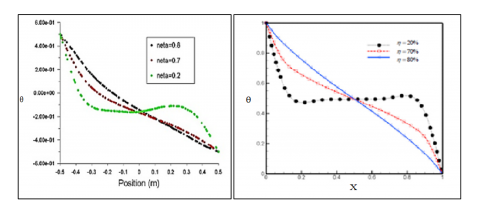Figure 8. Temperature profiles for different values of η as a function of the position y;

(a) Present study, (b) F. Habbechi 

The Nusselt number is inversely proportional to the increase of η. It tends to a constant value, when the thickness of the porous layer approaches zero corresponding to pure natural convection. Similarly, as the thickness of the porous media approaches unity, the flow tends to a purely conductive situation through the body. While the intermediate zone is characterized by a sharp decrease in the number of Nusselt as a function of η according to the following correlation: Nucorrelation=-17*η+14.71.

## 9.pngFigure 9. Mean Nusselt profiles as a function of (η),

for Ra = 105, Da = 10-2;

(a) Present study, (b) F. Habbechi 

3.3 Heat transfer effect: Nu as a function of Darcy (Da)

The variation of the permeability of the porous layer, the variation of the Darcy number, and the influences on heat transfer have been highlighted. The increase in the number of Darcy makes it possible to have much more the presence of the thermal and dynamic fields inside the porous layer. This means that when the permeability increases, we approach the fluid medium (cavity differentially heated).

## 10.png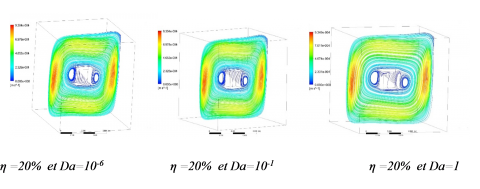Figure 10. Lines currents for different numbers of Da

The results show that for low values of permeability (Da≥10-6), the Nusselt numbers remain virtually constant, the porous medium in this Darcy range behaves like an impervious area where the flow is almost negligible. That is, conduction is dominant over natural convection. For the Darcy values between:(10-6 ≤ Da ≤ 10-1), there is an increase of Nusselt, and in this zone the flow tends towards a purely convective situation through the body, and for the high values of the permeability (Da ≥10-1), the heat transfer increases further up to the value of Nu=3.35 where the porous medium is considered as a fluid medium.

## 11.pngFigure 11. Evolution of the number of Nusselt according to Darcy (Da), for Ra = 105, η = 20% and η = 50%

4. Conclusion

The numerical study of natural convection heat transfer in a porous cubic cavity containing a fluid in a laminar regime was carried out. The left and right walls of the enclosure are heated and cooled, respectively, while the upper and lower walls are maintained adiabatic. The effects of the Rayleigh number, the Darcy number, the adimensional number of the porous layer thickness and the Nusselt number on heat transfer were considered.

This study is carried out by the "fluent" code of calculation allowing to solve the governing equations of the flow with transfer of heat by natural convection in a porous media. The flow field is governed by the Navier-Stokes equation in the fluid region and the Darcy-Brinkman-Forchheimer equation in the porous region. The thermal field is governed by the energy equation.

This study concluded that:

Thermal transfers increase with the number of Rayleigh, and this increase is all the more important as the permeability increases. The thermal transfer takes place essentially by conduction at (Ra=103) and with the increase of Rayleigh the natural convection will be dominant.

- The presence of a porous layer produces a sharp drop in transfers. This decrease is even lower when the permeability increases. For high values of permeability, the flow structure as well as the heat transfer are no longer sensitive to the thickness of the porous layer.

- The increase in the number of Darcy makes it possible to have much more the presence of the thermal and dynamic fields inside the porous layer. This means that as the permeability increases, we approach the fluid medium (differentially heated cavity).

Nomenclature

Latin letter

$\overrightarrow{V}$            Darcy velocity or filtration velocity (m / s);

P             Averaged magnitude of the pressure (Pa)

T             Averaged magnitude of the temperature (s);

Kf            Thermal conductivity of the fluid medium (W / m.K);

Ks            Thermal conductivity of the solid matrix (w / m.k);

Keff          Effective thermal conductivity (w / m.k);

g              Gravitational acceleration (m / s2);

F               Empirical factor which depends on the porosity and microstructure of the porous medium(-)

(X Y Z)     dimensionless coordinate system (-);

(U, V, W) Component dimensionless speed (-);

Greek symbols

ε             Porosity of the porous medium (-);

ρ0          Referential density (kg / m3);

ρf           Volumetric mass of the fluid (kg / m3);

μ            Dynamic viscosity of the fluid (kg / ms);

μeff        Dynamic viscosity of the porous medium (kg / ms);

σ            Report of specific heats of the equivalent medium and fluid (-);

Thermal conductivity of equivalent medium (-);

η            Thickness dimension without the porous layer (-);

Θ           Dimensionless temperature (-);

∆T         Variance of temperature (S);

β            Coefficient of thermal volume expansion of the fluid (k-1).

Indices

eff    Effective

f       Fluid

S      Solid

References

 Sami Ben Amara. (2005). Flows and heat transfer in natural convection in environments Macro-porous food application to household refrigerators. Physics [physics]. INAPG (AgroParisTech), 2005. English. <NNT: 2005INAP0006>. <Pastel-00001581>

 Ould-Amer Y, Slama S. (2007). Natural convection in a multilayer porous medium. J. Jacques, B. JITH 2007, Aug. 2007, Albi, France. ENSTIMAC, 5.

 Rebhi R, Alliche M, Mamou M. (2016). Numerical and analytical study of natural convection heat transfer in porous layers. NRC Publications Archive, NRC Publications Archive.

 Habbachi F, Oueslati FS, Bennacer R, Ganaoui M, Elcafsia A. (2017). Three-dimensional simulation of natural convection in cubic cavity. Partly filled with porous media. Energy Procedia 139: 617-623. https://doi.org/10.1016/j.egypro.2017.11.262

 Le Breton P, Caltagirone JP, Arquis E. (1991). Natural convection in a square cavity with thin porous layers on its vertical walls. J. Heat Transfer 113(4): 892-898. https://doi.org/10.1115/1.2911218